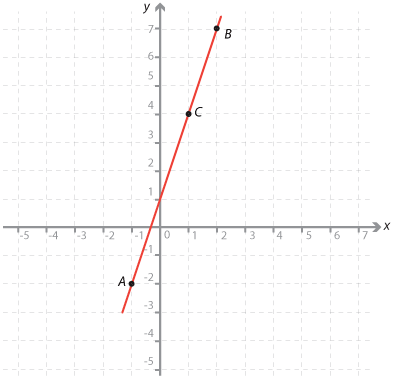#### Question 4

The points (–1, –2) and (2, 7) are plotted and the line is drawn through them.

The line has equation y = 3x + 1.Detailed description

 The point C(a, b) lies on the line. The equation relating a and b is: b = 3 × a + 1 a = 3 × b + 1 b = a + 1